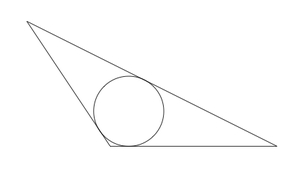# Circumscribed Triangles

Alignments to Content Standards: G-C.A.3

Below is a picture of a triangle circumscribed about a circle:Suppose we are given a circle $X$ and 3 points on the circle, $P$, $Q$, and $R$. When is there a triangle $ABC$ circumscribed about $X$, tangent to $X$ at $P$, $Q$, and $R$? Explain.

## IM Commentary

The goal of this task is to study where a circumscribed triangle can meet a given circle. Students will need to experiment and the teacher may need to provide guidance about the different configurations of the three points. The task is ideally suited for group work, allowing students to compare their constructions and divide up the many possible cases that need to be treated.

In the solution presented, the points where the triangle touches the circle are considered successively to make the logic of the construction as clear as possible. Students may require guidance if this type of approach is desired. It would be beneficial, however, to allow them to experiment first with different configurations before providing any additional instructions. This task is ideally suited for computer technology which would allow students to vary $P$, $Q$, and $R$ and explore what happens with the tangent lines at those three points.

## Solution

We will add each side of our circumscribed triangle separately to see what constraints there are. For the first side, we place the point $A$ of tangency with the circle at the top as shown in the picture below:For out second point $B$ of tangency, we have to avoid the point $A^\prime$ opposite $A$ as the tangent line to the circle at $A^\prime$ is parallel to the first line $\ell$. The point $B$ could be at any other point and we have drawn an example in the picture below with the tangent line to the circle at $B$ labeled $m$:For the third point $C$, there are multiple constraints. It cannot be opposite $A$ or $B$ for the reason described above. The points which do give a triangle circumscribing the circle are plotted below in green:For any point $C$ between $A^\prime$ and $B^\prime$ we find a triangle circumscribed about the circle. If $C$ is any other point, then $\ell$, $m$, and the line $k$ tangent to the circle at $C$ do not make a triangle circumscribed about the circle. For example, if $C$ is chosen between $B^\prime$ and $A$ (going clockwise) then $\ell$, $k$, and $m$ make a triangle but a triangle that is only tangent to the circle at $A$: the other points of tangency of the lines with the circle lie outside the triangle. All other cases can be treated similarly. Summing this up, given three points $A$, $B$, and $C$ on a circle, the circle can be inscribed in a triangle, tangent to the circle at $A$, $B$, and $C$, provided

• $B$ is not opposite $A$
• $\overset{\frown}{AB}$ measures less than 180$^\circ$ and $C$ lies on $\overset{\frown}{A^\prime B^\prime}$ (not at either endpoint of this arc).

Note in this second condition the arc $\overset{\frown}{A^\prime B^\prime}$ must be traversed in the same way (clockwise or counterclockwise) as $\overset{\frown}{AB}$.